## The 10 Algorithms Machine Learning Engineers Need to Know

Source: from kdnuggets.com (Good posts sometimes disappear, so I repost it here for my and for your information.)

Read this introductory list of contemporary machine learning algorithms of importance that every engineer should understand.

By James Le, New Story Charity.It is no doubt that the sub-field of machine learning / artificial intelligence has increasingly gained more popularity in the past couple of years. As Big Data is the hottest trend in the tech industry at the moment, machine learning is incredibly powerful to make predictions or calculated suggestions based on large amounts of data. Some of the most common examples of machine learning are Netflix’s algorithms to make movie suggestions based on movies you have watched in the past or Amazon’s algorithms that recommend books based on books you have bought before.

So if you want to learn more about machine learning, how do you start? For me, my first introduction is when I took an Artificial Intelligence class when I was studying abroad in Copenhagen. My lecturer is a full-time Applied Math and CS professor at the Technical University of Denmark, in which his research areas are logic and artificial, focusing primarily on the use of logic to model human-like planning, reasoning and problem solving. The class was a mix of discussion of theory/core concepts and hands-on problem solving. The textbook that we used is one of the AI classics: Peter Norvig’s Artificial Intelligence — A Modern Approach, in which we covered major topics including intelligent agents, problem-solving by searching, adversarial search, probability theory, multi-agent systems, social AI, philosophy/ethics/future of AI. At the end of the class, in a team of 3, we implemented simple search-based agents solving transportation tasks in a virtual environment as a programming project.

I have learned a tremendous amount of knowledge thanks to that class, and decided to keep learning about this specialized topic. In the last few weeks, I have been multiple tech talks in San Francisco on deep learning, neural networks, data architecture — and a Machine Learning conference with a lot of well-known professionals in the field. Most importantly, I enrolled inUdacity’s Intro to Machine Learning online course in the beginning of June and has just finished it a few days ago. In this post, I want to share some of the most common machine learning algorithms that I learned from the course.

Machine learning algorithms can be divided into 3 broad categories — supervised learning, unsupervised learning, and reinforcement learning.Supervised learning is useful in cases where a property (label) is available for a certain dataset (training set), but is missing and needs to be predicted for other instances. Unsupervised learning is useful in cases where the challenge is to discover implicit relationships in a given unlabeled dataset (items are not pre-assigned). Reinforcement learning falls between these 2 extremes — there is some form of feedback available for each predictive step or action, but no precise label or error message. Since this is an intro class, I didn’t learn about reinforcement learning, but I hope that 10 algorithms on supervised and unsupervised learning will be enough to keep you interested.

### Supervised Learning

1. Decision Trees: A decision tree is a decision support tool that uses a tree-like graph or model of decisions and their possible consequences, including chance-event outcomes, resource costs, and utility. Take a look at the image to get a sense of how it looks like.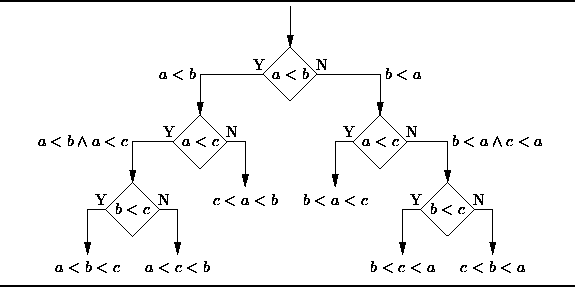Decision Tree
From a business decision point of view, a decision tree is the minimum number of yes/no questions that one has to ask, to assess the probability of making a correct decision, most of the time. As a method, it allows you to approach the problem in a structured and systematic way to arrive at a logical conclusion.

2. Naïve Bayes Classification: Naïve Bayes classifiers are a family of simple probabilistic classifiers based on applying Bayes’ theorem with strong (naïve) independence assumptions between the features. The featured image is the equation — with P(A|B) is posterior probability, P(B|A) is likelihood, P(A) is class prior probability, and P(B) is predictor prior probability.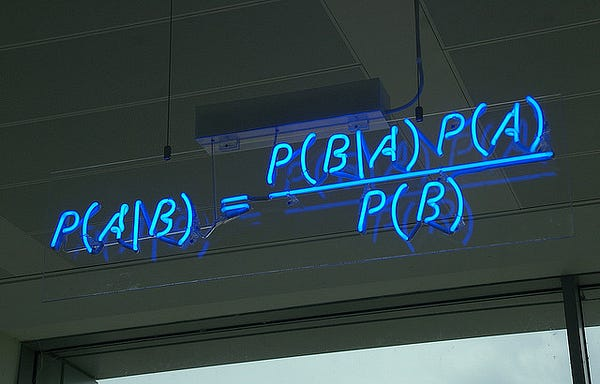Naive Bayes Classification
Some of real world examples are:

• To mark an email as spam or not spam
• Classify a news article about technology, politics, or sports
• Check a piece of text expressing positive emotions, or negative emotions?
• Used for face recognition software.

3. Ordinary Least Squares Regression: If you know statistics, you probably have heard of linear regression before. Least squares is a method for performing linear regression. You can think of linear regression as the task of fitting a straight line through a set of points. There are multiple possible strategies to do this, and “ordinary least squares” strategy go like this — You can draw a line, and then for each of the data points, measure the vertical distance between the point and the line, and add these up; the fitted line would be the one where this sum of distances is as small as possible.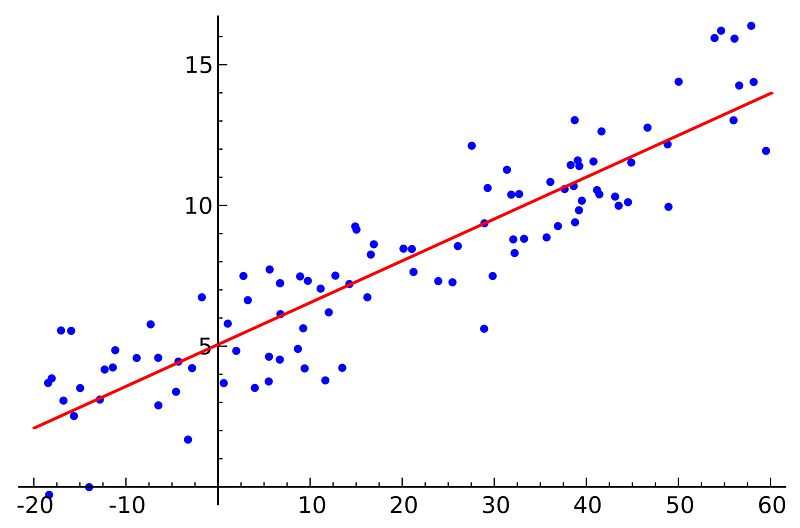Ordinary Least Squares Regression
Linear refers the kind of model you are using to fit the data, while least squares refers to the kind of error metric you are minimizing over.

4. Logistic Regression: Logistic regression is a powerful statistical way of modeling a binomial outcome with one or more explanatory variables. It measures the relationship between the categorical dependent variable and one or more independent variables by estimating probabilities using a logistic function, which is the cumulative logistic distribution.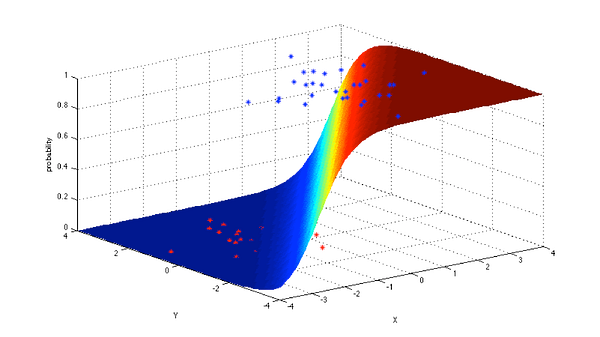Logistic Regression
In general, regressions can be used in real-world applications such as:

• Credit Scoring
• Measuring the success rates of marketing campaigns
• Predicting the revenues of a certain product
• Is there going to be an earthquake on a particular day?

5. Support Vector Machines: SVM is binary classification algorithm. Given a set of points of 2 types in N dimensional place, SVM generates a (N — 1) dimensional hyperlane to separate those points into 2 groups. Say you have some points of 2 types in a paper which are linearly separable. SVM will find a straight line which separates those points into 2 types and situated as far as possible from all those points.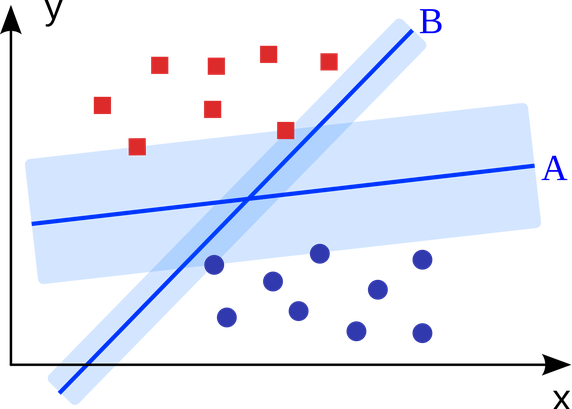Support Vector Machine
In terms of scale, some of the biggest problems that have been solved using SVMs (with suitably modified implementations) are display advertising, human splice site recognition, image-based gender detection, large-scale image classification…

6. Ensemble Methods: Ensemble methods are learning algorithms that construct a set of classifiers and then classify new data points by taking a weighted vote of their predictions. The original ensemble method is Bayesian averaging, but more recent algorithms include error-correcting output coding, bagging, and boosting.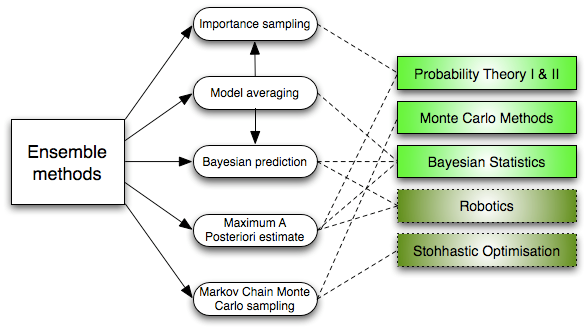Ensemble Learning Algorithms
So how do ensemble methods work and why are they superior to individual models?

• They average out biases: If you average a bunch of democratic-leaning polls and republican-leaning polls together, you will get an average something that isn’t leaning either way.
• They reduce the variance: The aggregate opinion of a bunch of models is less noisy than the single opinion of one of the models. In finance, this is called diversification — a mixed portfolio of many stocks will be much less variable than just one of the stocks alone. This is why your models will be better with more data points rather than fewer.
• They are unlikely to over-fit: If you have individual models that didn’t over-fit, and you are combining the predictions from each model in a simple way (average, weighted average, logistic regression), then there’s no room for over-fitting.

### Unsupervised Learning

7. Clustering Algorithms: Clustering is the task of grouping a set of objects such that objects in the same group (cluster) are more similar to each other than to those in other groups.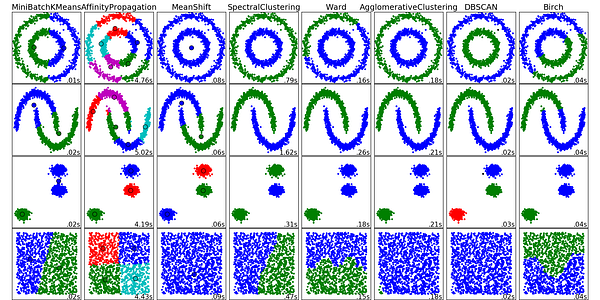Clustering Algorithms
Every clustering algorithm is different, and here are a couple of them:

• Centroid-based algorithms
• Connectivity-based algorithms
• Density-based algorithms
• Probabilistic
• Dimensionality Reduction
• Neural networks / Deep Learning

8. Principal Component Analysis: PCA is a statistical procedure that uses an orthogonal transformation to convert a set of observations of possibly correlated variables into a set of values of linearly uncorrelated variables called principal components.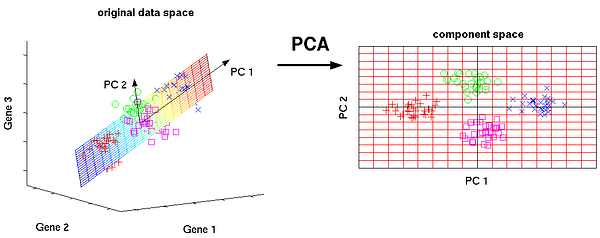Principal Component Analysis
Some of the applications of PCA include compression, simplifying data for easier learning, visualization. Notice that domain knowledge is very important while choosing whether to go forward with PCA or not. It is not suitable in cases where data is noisy (all the components of PCA have quite a high variance).

9. Singular Value Decomposition: In linear algebra, SVD is a factorization of a real complex matrix. For a given m * n matrix M, there exists a decomposition such that M = UΣV, where U and V are unitary matrices and Σ is a diagonal matrix.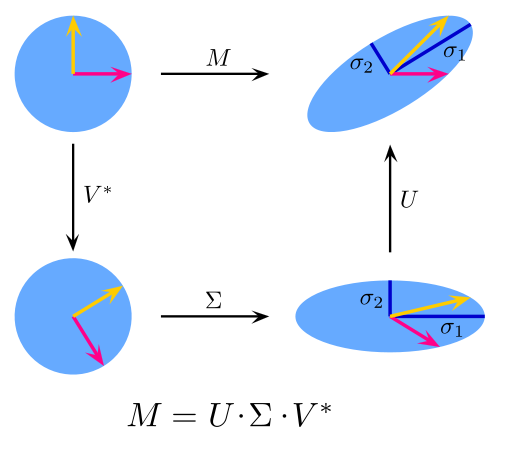Singular Value Decomposition
PCA is actually a simple application of SVD. In computer vision, the 1st face recognition algorithms used PCA and SVD in order to represent faces as a linear combination of “eigenfaces”, do dimensionality reduction, and then match faces to identities via simple methods; although modern methods are much more sophisticated, many still depend on similar techniques.

10. Independent Component Analysis: ICA is a statistical technique for revealing hidden factors that underlie sets of random variables, measurements, or signals. ICA defines a generative model for the observed multivariate data, which is typically given as a large database of samples. In the model, the data variables are assumed to be linear mixtures of some unknown latent variables, and the mixing system is also unknown. The latent variables are assumed non-gaussian and mutually independent, and they are called independent components of the observed data.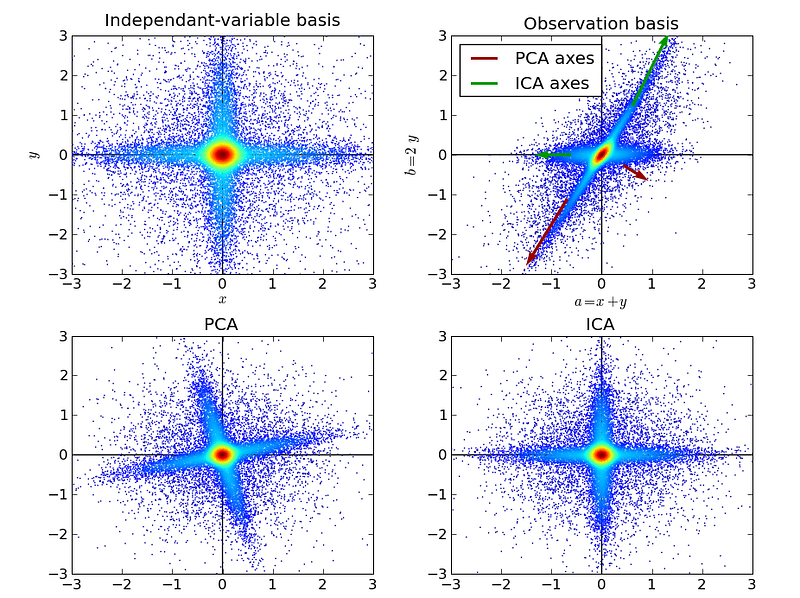Independent Component Analysis
ICA is related to PCA, but it is a much more powerful technique that is capable of finding the underlying factors of sources when these classic methods fail completely. Its applications include digital images, document databases, economic indicators and psychometric measurements.

Now go forth and wield your understanding of algorithms to create machine learning applications that make better experiences for people everywhere.

## Top 10 Data Mining Algorithms, Explained

source: from kdnuggets.com (Good posts sometimes disappear, so I repost it here for my and for your information.)

Top 10 data mining algorithms, selected by top researchers, are explained here, including what do they do, the intuition behind the algorithm, available implementations of the algorithms, why use them, and interesting applications.

By Raymond Li.

Today, I’m going to explain in plain English the top 10 most influential data mining algorithms as voted on by 3 separate panels in this survey paper.

Once you know what they are, how they work, what they do and where you can find them, my hope is you’ll have this blog post as a springboard to learn even more about data mining.

What are we waiting for? Let’s get started!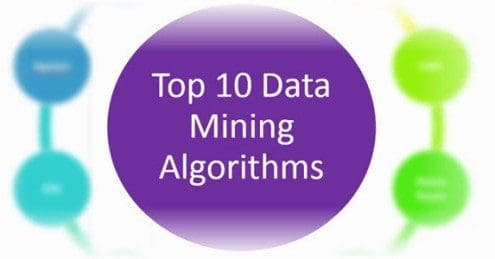Here are the algorithms:

• 1. C4.5
• 2. k-means
• 3. Support vector machines
• 4. Apriori
• 5. EM
• 6. PageRank
• 7. AdaBoost
• 8. kNN
• 9. Naive Bayes
• 10. CART

We also provide interesting resources at the end.

### 1. C4.5

What does it do? C4.5 constructs a classifier in the form of a decision tree. In order to do this, C4.5 is given a set of data representing things that are already classified.

Wait, what’s a classifier? A classifier is a tool in data mining that takes a bunch of data representing things we want to classify and attempts to predict which class the new data belongs to.

What’s an example of this? Sure, suppose a dataset contains a bunch of patients. We know various things about each patient like age, pulse, blood pressure, VO2max, family history, etc. These are called attributes.

Now:

Given these attributes, we want to predict whether the patient will get cancer. The patient can fall into 1 of 2 classes: will get cancer or won’t get cancer. C4.5 is told the class for each patient.

And here’s the deal:

Using a set of patient attributes and the patient’s corresponding class, C4.5 constructs a decision tree that can predict the class for new patients based on their attributes.

Cool, so what’s a decision tree? Decision tree learning creates something similar to a flowchart to classify new data. Using the same patient example, one particular path in the flowchart could be:

• Patient has a history of cancer
• Patient is expressing a gene highly correlated with cancer patients
• Patient has tumors
• Patient’s tumor size is greater than 5cm

The bottom line is:

At each point in the flowchart is a question about the value of some attribute, and depending on those values, he or she gets classified. You can find lots of examples of decision trees.

Is this supervised or unsupervised? This is supervised learning, since the training dataset is labeled with classes. Using the patient example, C4.5 doesn’t learn on its own that a patient will get cancer or won’t get cancer. We told it first, it generated a decision tree, and now it uses the decision tree to classify.

You might be wondering how C4.5 is different than other decision tree systems?

• First, C4.5 uses information gain when generating the decision tree.
• Second, although other systems also incorporate pruning, C4.5 uses a single-pass pruning process to mitigate over-fitting. Pruning results in many improvements.
• Third, C4.5 can work with both continuous and discrete data. My understanding is it does this by specifying ranges or thresholds for continuous data thus turning continuous data into discrete data.
• Finally, incomplete data is dealt with in its own ways.

Why use C4.5? Arguably, the best selling point of decision trees is their ease of interpretation and explanation. They are also quite fast, quite popular and the output is human readable.

Where is it used? A popular open-source Java implementation can be found over at OpenTox. Orange, an open-source data visualization and analysis tool for data mining, implements C4.5 in their decision tree classifier.

Classifiers are great, but make sure to checkout the next algorithm about clustering…

### 2. k-means

What does it do? k-means creates k groups from a set of objects so that the members of a group are more similar. It’s a popular cluster analysis technique for exploring a dataset.

Hang on, what’s cluster analysis? Cluster analysis is a family of algorithms designed to form groups such that the group members are more similar versus non-group members. Clusters and groups are synonymous in the world of cluster analysis.

Is there an example of this? Definitely, suppose we have a dataset of patients. In cluster analysis, these would be called observations. We know various things about each patient like age, pulse, blood pressure, VO2max, cholesterol, etc. This is a vector representing the patient.

Look:

You can basically think of a vector as a list of numbers we know about the patient. This list can also be interpreted as coordinates in multi-dimensional space. Pulse can be one dimension, blood pressure another dimension and so forth.

You might be wondering:

Given this set of vectors, how do we cluster together patients that have similar age, pulse, blood pressure, etc?

Want to know the best part?

You tell k-means how many clusters you want. K-means takes care of the rest.

How does k-means take care of the rest? k-means has lots of variations to optimize for certain types of data.

At a high level, they all do something like this:

1. k-means picks points in multi-dimensional space to represent each of the k clusters. These are called centroids.
2. Every patient will be closest to 1 of these k centroids. They hopefully won’t all be closest to the same one, so they’ll form a cluster around their nearest centroid.
3. What we have are k clusters, and each patient is now a member of a cluster.
4. k-means then finds the center for each of the k clusters based on its cluster members (yep, using the patient vectors!).
5. This center becomes the new centroid for the cluster.
6. Since the centroid is in a different place now, patients might now be closer to other centroids. In other words, they may change cluster membership.
7. Steps 2-6 are repeated until the centroids no longer change, and the cluster memberships stabilize. This is called convergence.

Is this supervised or unsupervised? It depends, but most would classify k-means as unsupervised. Other than specifying the number of clusters, k-means “learns” the clusters on its own without any information about which cluster an observation belongs to. k-means can be semi-supervised.

Why use k-means? I don’t think many will have an issue with this:

The key selling point of k-means is its simplicity. Its simplicity means it’s generally faster and more efficient than other algorithms, especially over large datasets.

It gets better:

k-means can be used to pre-cluster a massive dataset followed by a more expensive cluster analysis on the sub-clusters. k-means can also be used to rapidly “play” with k and explore whether there are overlooked patterns or relationships in the dataset.

It’s not all smooth sailing:

Two key weaknesses of k-means are its sensitivity to outliers, and its sensitivity to the initial choice of centroids. One final thing to keep in mind is k-means is designed to operate on continuous data — you’ll need to do some tricks to get it to work on discrete data.

Where is it used? A ton of implementations for k-means clustering are available online:

If decision trees and clustering didn’t impress you, you’re going to love the next algorithm.

### 3. Support vector machines

What does it do? Support vector machine (SVM) learns a hyperplane to classify data into 2 classes. At a high-level, SVM performs a similar task like C4.5 except SVM doesn’t use decision trees at all.

Whoa, a hyper-what? A hyperplane is a function like the equation for a line, y = mx + b. In fact, for a simple classification task with just 2 features, the hyperplane can be a line.

As it turns out…

SVM can perform a trick to project your data into higher dimensions. Once projected into higher dimensions…

…SVM figures out the best hyperplane which separates your data into the 2 classes.

Do you have an example? Absolutely, the simplest example I found starts with a bunch of red and blue balls on a table. If the balls aren’t too mixed together, you could take a stick and without moving the balls, separate them with the stick.

You see:

When a new ball is added on the table, by knowing which side of the stick the ball is on, you can predict its color.

What do the balls, table and stick represent? The balls represent data points, and the red and blue color represent 2 classes. The stick represents the simplest hyperplane which is a line.

And the coolest part?

SVM figures out the function for the hyperplane.

What if things get more complicated? Right, they frequently do. If the balls are mixed together, a straight stick won’t work.

Here’s the work-around:

Quickly lift up the table throwing the balls in the air. While the balls are in the air and thrown up in just the right way, you use a large sheet of paper to divide the balls in the air.

You might be wondering if this is cheating:

Nope, lifting up the table is the equivalent of mapping your data into higher dimensions. In this case, we go from the 2 dimensional table surface to the 3 dimensional balls in the air.

How does SVM do this? By using a kernel we have a nice way to operate in higher dimensions. The large sheet of paper is still called a hyperplane, but it is now a function for a plane rather than a line. Note from Yuval that once we’re in 3 dimensions, the hyperplane must be a plane rather than a line.

I found this visualization super helpful:

Reddit also has 2 great threads on this in the ELI5 and ML subreddits.

How do balls on a table or in the air map to real-life data? A ball on a table has a location that we can specify using coordinates. For example, a ball could be 20cm from the left edge and 50cm from the bottom edge. Another way to describe the ball is as (x, y) coordinates or (20, 50). x and y are 2 dimensions of the ball.

Here’s the deal:

If we had a patient dataset, each patient could be described by various measurements like pulse, cholesterol level, blood pressure, etc. Each of these measurements is a dimension.

The bottom line is:

SVM does its thing, maps them into a higher dimension and then finds the hyperplane to separate the classes.

Margins are often associated with SVM? What are they? The margin is the distance between the hyperplane and the 2 closest data points from each respective class. In the ball and table example, the distance between the stick and the closest red and blue ball is the margin.

The key is:

SVM attempts to maximize the margin, so that the hyperplane is just as far away from red ball as the blue ball. In this way, it decreases the chance of misclassification.

Where does SVM get its name from? Using the ball and table example, the hyperplane is equidistant from a red ball and a blue ball. These balls or data points are called support vectors, because they support the hyperplane.

Is this supervised or unsupervised? This is a supervised learning, since a dataset is used to first teach the SVM about the classes. Only then is the SVM capable of classifying new data.

Why use SVM? SVM along with C4.5 are generally the 2 classifiers to try first. No classifier will be the best in all cases due to the No Free Lunch Theorem. In addition, kernel selection and interpretability are some weaknesses.

Where is it used? There are many implementations of SVM. A few of the popular ones are scikit-learn, MATLAB and of course libsvm.

The next algorithm is one of my favorites…

### 4. Apriori

What does it do? The Apriori algorithm learns association rules and is applied to a database containing a large number of transactions.

What are association rules? Association rule learning is a data mining technique for learning correlations and relations among variables in a database.

What’s an example of Apriori? Let’s say we have a database full of supermarket transactions. You can think of a database as a giant spreadsheet where each row is a customer transaction and every column represents a different grocery item.Here’s the best part:

By applying the Apriori algorithm, we can learn the grocery items that are purchased together a.k.a association rules.

The power of this is:

You can find those items that tend to be purchased together more frequently than other items — the ultimate goal being to get shoppers to buy more. Together, these items are called itemsets.

For example:

You can probably quickly see that chips + dip and chips + soda seem to frequently occur together. These are called 2-itemsets. With a large enough dataset, it will be much harder to “see” the relationships especially when you’re dealing with 3-itemsets or more. That’s precisely what Apriori helps with!

You might be wondering how Apriori works? Before getting into the nitty-gritty of algorithm, you’ll need to define 3 things:

1. The first is the size of your itemset. Do you want to see patterns for a 2-itemset, 3-itemset, etc.?
2. The second is your support or the number of transactions containing the itemset divided by the total number of transactions. An itemset that meets the support is called a frequent itemset.
3. The third is your confidence or the conditional probability of some item given you have certain other items in your itemset. A good example is given chips in your itemset, there is a 67% confidence of having soda also in the itemset.

The basic Apriori algorithm is a 3 step approach:

1. Join. Scan the whole database for how frequent 1-itemsets are.
2. Prune. Those itemsets that satisfy the support and confidence move onto the next round for 2-itemsets.
3. Repeat. This is repeated for each itemset level until we reach our previously defined size.

Is this supervised or unsupervised? Apriori is generally considered an unsupervised learning approach, since it’s often used to discover or mine for interesting patterns and relationships.

But wait, there’s more…

Apriori can also be modified to do classification based on labelled data.

Why use Apriori? Apriori is well understood, easy to implement and has many derivatives.

On the other hand…

The algorithm can be quite memory, space and time intensive when generating itemsets.

Where is it used? Plenty of implementations of Apriori are available. Some popular ones are the ARtool, Weka, and Orange.

The next algorithm was the most difficult for me to understand, look at the next algorithm…

### 5. EM

What does it do? In data mining, expectation-maximization (EM) is generally used as a clustering algorithm (like k-means) for knowledge discovery.

In statistics, the EM algorithm iterates and optimizes the likelihood of seeing observed data while estimating the parameters of a statistical model with unobserved variables.

OK, hang on while I explain…

I’m not a statistician, so hopefully my simplification is both correct and helps with understanding.

Here are a few concepts that will make this way easier…

What’s a statistical model? I see a model as something that describes how observed data is generated. For example, the grades for an exam could fit a bell curve, so the assumption that the grades are generated via a bell curve (a.k.a. normal distribution) is the model.

Wait, what’s a distribution? A distribution represents the probabilities for all measurable outcomes. For example, the grades for an exam could fit a normal distribution. This normal distribution represents all the probabilities of a grade.

In other words, given a grade, you can use the distribution to determine how many exam takers are expected to get that grade.

Cool, what are the parameters of a model? A parameter describes a distribution which is part of a model. For example, a bell curve can be described by its mean and variance.

Using the exam scenario, the distribution of grades on an exam (the measurable outcomes) followed a bell curve (this is the distribution). The mean was 85 and the variance was 100.

So, all you need to describe a normal distribution are 2 parameters:

1. The mean
2. The variance

And likelihood? Going back to our previous bell curve example… suppose we have a bunch of grades and are told the grades follow a bell curve. However, we’re not given all the grades… only a sample.

Here’s the deal:

We don’t know the mean or variance of all the grades, but we can estimate them using the sample. The likelihood is the probability that the bell curve with estimated mean and variance results in those bunch of grades.

In other words, given a set of measurable outcomes, let’s estimate the parameters. Using these estimated parameters, the hypothetical probability of the outcomes is called likelihood.

Remember, it’s the hypothetical probability of the existing grades, not the probability of a future grade.

You’re probably wondering, what’s probability then?

Using the bell curve example, suppose we know the mean and variance. Then we’re told the grades follow a bell curve. The chance that we observe certain grades and how often they are observed is the probability.

In more general terms, given the parameters, let’s estimate what outcomes should be observed. That’s what probability does for us.

Great! Now, what’s the difference between observed and unobserved data? Observed data is the data that you saw or recorded. Unobserved data is data that is missing. There a number of reasons that the data could be missing (not recorded, ignored, etc.).

Here’s the kicker:

For data mining and clustering, what’s important to us is looking at the class of a data point as missing data. We don’t know the class, so interpreting missing data this way is crucial for applying EM to the task of clustering.

Once again: The EM algorithm iterates and optimizes the likelihood of seeing observed data while estimating the parameters of a statistical model with unobserved variables. Hopefully, this is way more understandable now.

The best part is…

By optimizing the likelihood, EM generates an awesome model that assigns class labels to data points — sounds like clustering to me!

How does EM help with clustering? EM begins by making a guess at the model parameters.

Then it follows an iterative 3-step process:

1. E-step: Based on the model parameters, it calculates the probabilities for assignments of each data point to a cluster.
2. M-step: Update the model parameters based on the cluster assignments from the E-step.
3. Repeat until the model parameters and cluster assignments stabilize (a.k.a. convergence).

Is this supervised or unsupervised? Since we do not provide labeled class information, this is unsupervised learning.

Why use EM? A key selling point of EM is it’s simple and straight-forward to implement. In addition, not only can it optimize for model parameters, it can also iteratively make guesses about missing data.

This makes it great for clustering and generating a model with parameters. Knowing the clusters and model parameters, it’s possible to reason about what the clusters have in common and which cluster new data belongs to.

EM is not without weaknesses though…

• First, EM is fast in the early iterations, but slow in the later iterations.
• Second, EM doesn’t always find the optimal parameters and gets stuck in local optima rather than global optima.

Where is it used? The EM algorithm is available in Weka. R has an implementation in the mclust package. scikit-learn also has an implementation in its gmm module.

What data mining does Google do? Take a look…

### 6. PageRank

What does it do? PageRank is a link analysis algorithm designed to determine the relative importance of some object linked within a network of objects.

Yikes.. what’s link analysis? It’s a type of network analysis looking to explore the associations (a.k.a. links) among objects.

Here’s an example: The most prevalent example of PageRank is Google’s search engine. Although their search engine doesn’t solely rely on PageRank, it’s one of the measures Google uses to determine a web page’s importance.

Let me explain:

Web pages on the World Wide Web link to each other. If rayli.net links to a web page on CNN, a vote is added for the CNN page indicating rayli.net finds the CNN web page relevant.

And it doesn’t stop there…

rayli.net’s votes are in turn weighted by rayli.net’s importance and relevance. In other words, any web page that’s voted for rayli.net increases rayli.net’s relevance.

The bottom line?

This concept of voting and relevance is PageRank. rayli.net’s vote for CNN increases CNN’s PageRank, and the strength of rayli.net’s PageRank influences how much its vote affects CNN’s PageRank.

What does a PageRank of 0, 1, 2, 3, etc. mean? Although the precise meaning of a PageRank number isn’t disclosed by Google, we can get a sense of its relative meaning.

And here’s how:You see?

It’s a bit like a popularity contest. We all have a sense of which websites are relevant and popular in our minds. PageRank is just an uber elegant way to define it.

What other applications are there of PageRank? PageRank was specifically designed for the World Wide Web.

Think about it:

At its core, PageRank is really just a super effective way to do link analysis.The objects being linked don’t have to be web pages.

Here are 3 innovative applications of PageRank:

1. Dr Stefano Allesina, from the University of Chicago, applied PageRank to ecology to determine which species are critical for sustaining ecosystems.
2. Twitter developed WTF (Who-to-Follow) which is a personalized PageRank recommendation engine about who to follow.
3. Bin Jiang, from The Hong Kong Polytechnic University, used a variant of PageRank to predict human movement rates based on topographical metrics in London.

Is this supervised or unsupervised? PageRank is generally considered an unsupervised learning approach, since it’s often used to discover the importance or relevance of a web page.

Why use PageRank? Arguably, the main selling point of PageRank is its robustness due to the difficulty of getting a relevant incoming link.

Simply stated:

If you have a graph or network and want to understand relative importance, priority, ranking or relevance, give PageRank a try.

Where is it used? The PageRank trademark is owned by Google. However, the PageRank algorithm is actually patented by Stanford University.

You might be wondering if you can use PageRank:

I’m not a lawyer, so best to check with an actual lawyer, but you can probably use the algorithm as long as it doesn’t commercially compete against Google/Stanford.

Here are 3 implementations of PageRank:

With our powers combined, we are…

### 7. AdaBoost

What does it do? AdaBoost is a boosting algorithm which constructs a classifier.

As you probably remember, a classifier takes a bunch of data and attempts to predict or classify which class a new data element belongs to.

But what’s boosting? Boosting is an ensemble learning algorithm which takes multiple learning algorithms (e.g. decision trees) and combines them. The goal is to take an ensemble or group of weak learners and combine them to create a single strong learner.

What’s the difference between a strong and weak learner? A weak learner classifies with accuracy barely above chance. A popular example of a weak learner is the decision stump which is a one-level decision tree.

Alternatively…

A strong learner has much higher accuracy, and an often used example of a strong learner is SVM.

What’s an example of AdaBoost? Let’s start with 3 weak learners. We’re going to train them in 10 rounds on a training dataset containing patient data. The dataset contains details about the patient’s medical records.

The question is…

How can we predict whether the patient will get cancer?

Here’s how AdaBoost answers the question…

In round 1: AdaBoost takes a sample of the training dataset and tests to see how accurate each learner is. The end result is we find the best learner.

In addition, samples that are misclassified are given a heavier weight, so that they have a higher chance of being picked in the next round.

One more thing, the best learner is also given a weight depending on its accuracy and incorporated into the ensemble of learners (right now there’s just 1 learner).

In round 2: AdaBoost again attempts to look for the best learner.

And here’s the kicker:

The sample of patient training data is now influenced by the more heavily misclassified weights. In other words, previously misclassified patients have a higher chance of showing up in the sample.

Why?

It’s like getting to the second level of a video game and not having to start all over again when your character is killed. Instead, you start at level 2 and focus all your efforts on getting to level 3.

Likewise, the first learner likely classified some patients correctly. Instead of trying to classify them again, let’s focus all the efforts on getting the misclassified patients.

The best learner is again weighted and incorporated into the ensemble, misclassified patients are weighted so they have a higher chance of being picked and we rinse and repeat.

At the end of the 10 rounds: We’re left with an ensemble of weighted learners trained and then repeatedly retrained on misclassified data from the previous rounds.

Is this supervised or unsupervised? This is supervised learning, since each iteration trains the weaker learners with the labelled dataset.

Why use AdaBoost? AdaBoost is simple. The algorithm is relatively straight-forward to program.

In addition, it’s fast! Weak learners are generally simpler than strong learners. Being simpler means they’ll likely execute faster.

Another thing…

It’s a super elegant way to auto-tune a classifier, since each successive AdaBoost round refines the weights for each of the best learners. All you need to specify is the number of rounds.

Finally, it’s flexible and versatile. AdaBoost can incorporate any learning algorithm, and it can work with a large variety of data.

Where is it used? AdaBoost has a ton of implementations and variants. Here are a few:

If you like Mr. Rogers, you’ll like the next algorithm…

### 8. kNN

What does it do? kNN, or k-Nearest Neighbors, is a classification algorithm. However, it differs from the classifiers previously described because it’s a lazy learner.

What’s a lazy learner? A lazy learner doesn’t do much during the training process other than store the training data. Only when new unlabeled data is input does this type of learner look to classify.

On the other hand, an eager learner builds a classification model during training. When new unlabeled data is input, this type of learner feeds the data into the classification model.

How does C4.5, SVM and AdaBoost fit into this? Unlike kNN, they are all eager learners.

Here’s why:

1. C4.5 builds a decision tree classification model during training.
2. SVM builds a hyperplane classification model during training.
3. AdaBoost builds an ensemble classification model during training.

So what does kNN do? kNN builds no such classification model. Instead, it just stores the labeled training data.

When new unlabeled data comes in, kNN operates in 2 basic steps:

1. First, it looks at the$k$ closest labeled training data points — in other words, the k-nearest neighbors.
2. Second, using the neighbors’ classes, kNN gets a better idea of how the new data should be classified.

You might be wondering…

How does kNN figure out what’s closer? For continuous data, kNN uses a distance metric like Euclidean distance. The choice of distance metric largely depends on the data. Some even suggest learning a distance metric based on the training data. There’s tons more details and papers on kNN distance metrics.

For discrete data, the idea is transform discrete data into continuous data. 2 examples of this are:

1. Using Hamming distance as a metric for the “closeness” of 2 text strings.
2. Transforming discrete data into binary features.

These 2 Stack Overflow threads have some more suggestions on dealing with discrete data:

How does kNN classify new data when neighbors disagree? kNN has an easy time when all neighbors are the same class. The intuition is if all the neighbors agree, then the new data point likely falls in the same class.

I’ll bet you can guess where things get hairy…

How does kNN decide the class when neighbors don’t have the same class?

2 common techniques for dealing with this are:

1. Take a simple majority vote from the neighbors. Whichever class has the greatest number of votes becomes the class for the new data point.
2. Take a similar vote except give a heavier weight to those neighbors that are closer. A simple way to do this is to use reciprocal distance e.g. if the neighbor is 5 units away, then weight its vote 1/5. As the neighbor gets further away, the reciprocal distance gets smaller and smaller… exactly what we want!

Is this supervised or unsupervised? This is supervised learning, since kNN is provided a labeled training dataset.

Why use kNN? Ease of understanding and implementing are 2 of the key reasons to use kNN. Depending on the distance metric, kNN can be quite accurate.

But that’s just part of the story…

Here are 5 things to watch out for:

1. kNN can get very computationally expensive when trying to determine the nearest neighbors on a large dataset.
2. Noisy data can throw off kNN classifications.
3. Features with a larger range of values can dominate the distance metric relative to features that have a smaller range, so feature scaling is important.
4. Since data processing is deferred, kNN generally requires greater storage requirements than eager classifiers.
5. Selecting a good distance metric is crucial to kNN’s accuracy.

Where is it used? A number of kNN implementations exist:

Spam? Fuhgeddaboudit! Read ahead to learn about the next algorithm…

### 9. Naive Bayes

What does it do? Naive Bayes is not a single algorithm, but a family of classification algorithms that share one common assumption:

Every feature of the data being classified is independent of all other features given the class.

What does independent mean? 2 features are independent when the value of one feature has no effect on the value of another feature.

For example:

Let’s say you have a patient dataset containing features like pulse, cholesterol level, weight, height and zip code. All features would be independent if the value of all features have no effect on each other. For this dataset, it’s reasonable to assume that the patient’s height and zip code are independent, since a patient’s height has little to do with their zip code.

But let’s not stop there, are the other features independent?

Sadly, the answer is no. Here are 3 feature relationships which are not independent:

• If height increases, weight likely increases.
• If cholesterol level increases, weight likely increases.
• If cholesterol level increases, pulse likely increases as well.

In my experience, the features of a dataset are generally not all independent.

And that ties in with the next question…

Why is it called naive? The assumption that all features of a dataset are independent is precisely why it’s called naive — it’s generally not the case that all features are independent.

What’s Bayes? Thomas Bayes was an English statistician for which Bayes’ Theorem is named after. You can click on the link to find about more about Bayes’ Theorem.

In a nutshell, the theorem allows us to predict the class given a set of features using probability.

The simplified equation for classification looks something like this:$P(\textit{Class A}|\textit{Feature 1}, \textit{Feature 2}) = \dfrac{P(\textit{Feature 1}|\textit{Class A}) \cdot P(\textit{Feature 2}|\textit{Class A}) \cdot P(\textit{Class A})}{P(\textit{Feature 1}) \cdot P(\textit{Feature 2})}$

Let’s dig deeper into this…

What does the equation mean? The equation finds the probability of Class A given Features 1 and 2. In other words, if you see Features 1 and 2, this is the probability the data is Class A.

The equation reads: The probability of Class A given Features 1 and 2 is a fraction.

• The fraction’s numerator is the probability of Feature 1 given Class A multiplied by the probability of Feature 2 given Class A multiplied by the probability of Class A.
• The fraction’s denominator is the probability of Feature 1 multiplied by the probability of Feature 2.

What is an example of Naive Bayes? Below is a great example taken from a Stack Overflow thread.

Here’s the deal:

• We have a training dataset of 1,000 fruits.
• The fruit can be a Banana, Orange or Other (these are the classes).
• The fruit can be Long, Sweet or Yellow (these are the features).What do you see in this training dataset?

• Out of 500 bananas, 400 are long, 350 are sweet and 450 are yellow.
• Out of 300 oranges, none are long, 150 are sweet and 300 are yellow.
• Out of the remaining 200 fruit, 100 are long, 150 are sweet and 50 are yellow.

If we are given the length, sweetness and color of a fruit (without knowing its class), we can now calculate the probability of it being a banana, orange or other fruit.

Suppose we are told the unknown fruit is long, sweet and yellow.

Here’s how we calculate all the probabilities in 4 steps:

Step 1: To calculate the probability the fruit is a banana, let’s first recognize that this looks familiar. It’s the probability of the class Banana given the features Long, Sweet and Yellow or more succinctly:$P(Banana|Long, Sweet, Yellow)$

This is exactly like the equation discussed earlier.

Step 2: Starting with the numerator, let’s plug everything in.

•$P(Long|Banana) = 400/500 = 0.8$
•$P(Sweet|Banana) = 350/500 = 0.7$
•$P(Yellow|Banana) = 450/500 = 0.9$
•$P(Banana) = 500 / 1000 = 0.5$

Multiplying everything together (as in the equation), we get:$0.8 \times 0.7 \times 0.9 \times 0.5 = 0.252$

Step 3: Ignore the denominator, since it’ll be the same for all the other calculations.

Step 4: Do a similar calculation for the other classes:

•$P(Orange|Long, Sweet, Yellow) = 0$
•$P(Other|Long, Sweet, Yellow) = 0.01875$

Since the$0.252$ is greater than$0.01875$, Naive Bayes would classify this long, sweet and yellow fruit as a banana.

Is this supervised or unsupervised? This is supervised learning, since Naive Bayes is provided a labeled training dataset in order to construct the tables.

Why use Naive Bayes? As you could see in the example above, Naive Bayes involves simple arithmetic. It’s just tallying up counts, multiplying and dividing.

Once the frequency tables are calculated, classifying an unknown fruit just involves calculating the probabilities for all the classes, and then choosing the highest probability.

Despite its simplicity, Naive Bayes can be surprisingly accurate. For example, it’s been found to be effective for spam filtering.

Where is it used? Implementations of Naive Bayes can be found in Orange, scikit-learn, Weka and R.

Finally, check out the 10th algorithm…

### 10. CART

What does it do? CART stands for classification and regression trees. It is a decision tree learning technique that outputs either classification or regression trees. Like C4.5, CART is a classifier.

Is a classification tree like a decision tree? A classification tree is a type of decision tree. The output of a classification tree is a class.

For example, given a patient dataset, you might attempt to predict whether the patient will get cancer. The class would either be “will get cancer” or “won’t get cancer.”

What’s a regression tree? Unlike a classification tree which predicts a class, regression trees predict a numeric or continuous value e.g. a patient’s length of stay or the price of a smartphone.

Here’s an easy way to remember…

Classification trees output classes, regression trees output numbers.

Since we’ve already covered how decision trees are used to classify data, let’s jump right into things…

How does this compare with C4.5?

C4.5 CART
Uses information gain to segment data during decision tree generation. Uses Gini impurity (not to be confused with Gini coefficient). A good discussion of the differences between the impurity and coefficient is available on Stack Overflow.
Uses a single-pass pruning process to mitigate over-fitting. Uses the cost-complexity method of pruning. Starting at the bottom of the tree, CART evaluates the misclassification cost with the node vs. without the node. If the cost doesn’t meet a threshold, it is pruned away.
The decision nodes can have 2 or more branches. The decision nodes have exactly 2 branches.
Probabilistically distributes missing values to children. Uses surrogates to distribute the missing values to children.

Is this supervised or unsupervised? CART is a supervised learning technique, since it is provided a labeled training dataset in order to construct the classification or regression tree model.

Why use CART? Many of the reasons you’d use C4.5 also apply to CART, since they are both decision tree learning techniques. Things like ease of interpretation and explanation also apply to CART as well.

Like C4.5, they are also quite fast, quite popular and the output is human readable.

Where is it used? scikit-learn implements CART in their decision tree classifier. R’s tree package has an implementation of CART. Weka and MATLAB also have implementations.

## Great talks about Machine Learning and Deep Learning

(Stay tuned in, the list is growing over time)